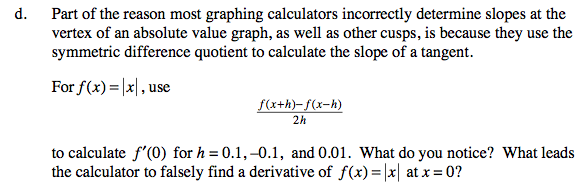### Home > CALC > Chapter 3 > Lesson 3.4.4 > Problem3-188

3-188.
1. ANOTHER PROBLEM FOR HANAH Homework Help ✎

1. Graph y = x2/3 on graph paper and without your calculator, sketch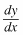.

2. What happens toat the vertex?

3. Use your graphing calculator to find the slope of y = x2/3 at the vertex. What happened?

4. Part of the reason most graphing calculators incorrectly determine slopes at the vertex of an absolute value graph, as well as other cusps, is because they use the symmetric difference quotient to calculate the slope of a tangent.

For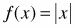, use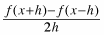to calculate f ′(−0) for h = 0.1, −0.1, and 0.01. What do you notice? What leads the calculator to falsely find a derivative ofat x = 0?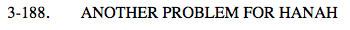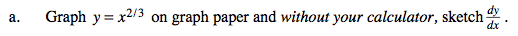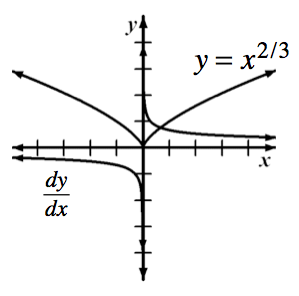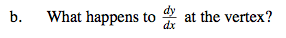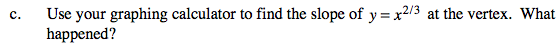Though the derivative does not exist, some graphing calculators (inaccurately) say that the derivative is 0 at a cusp.